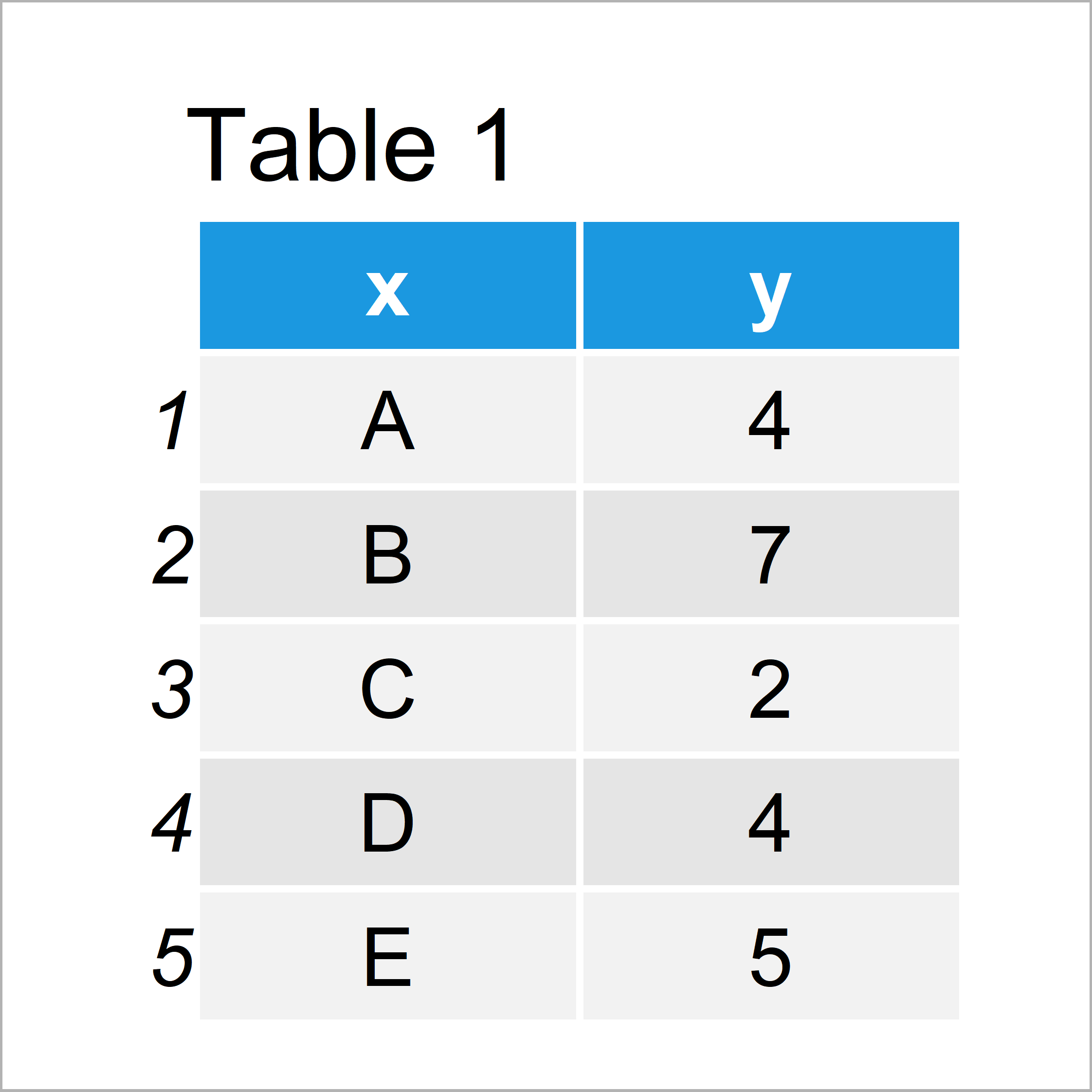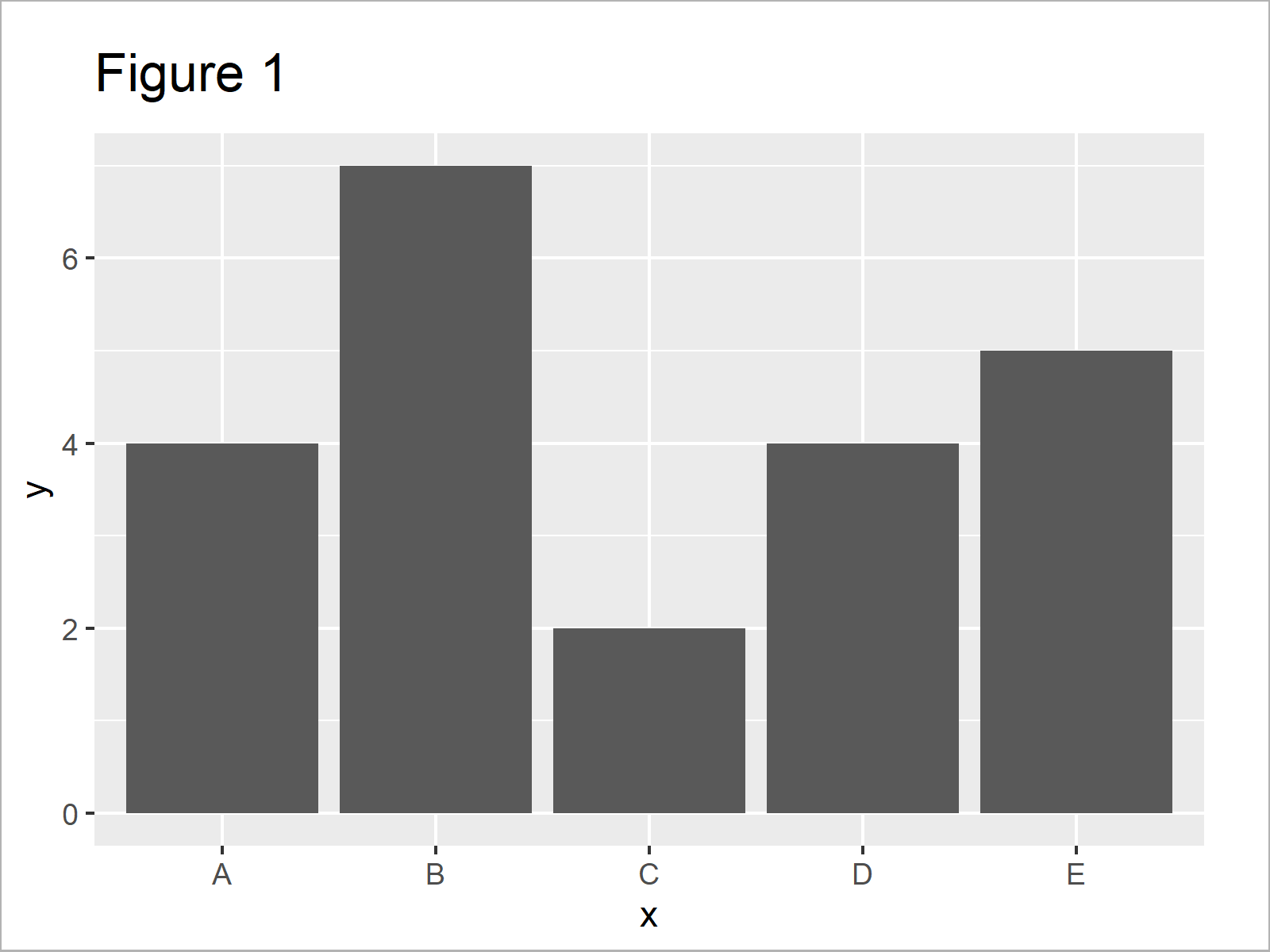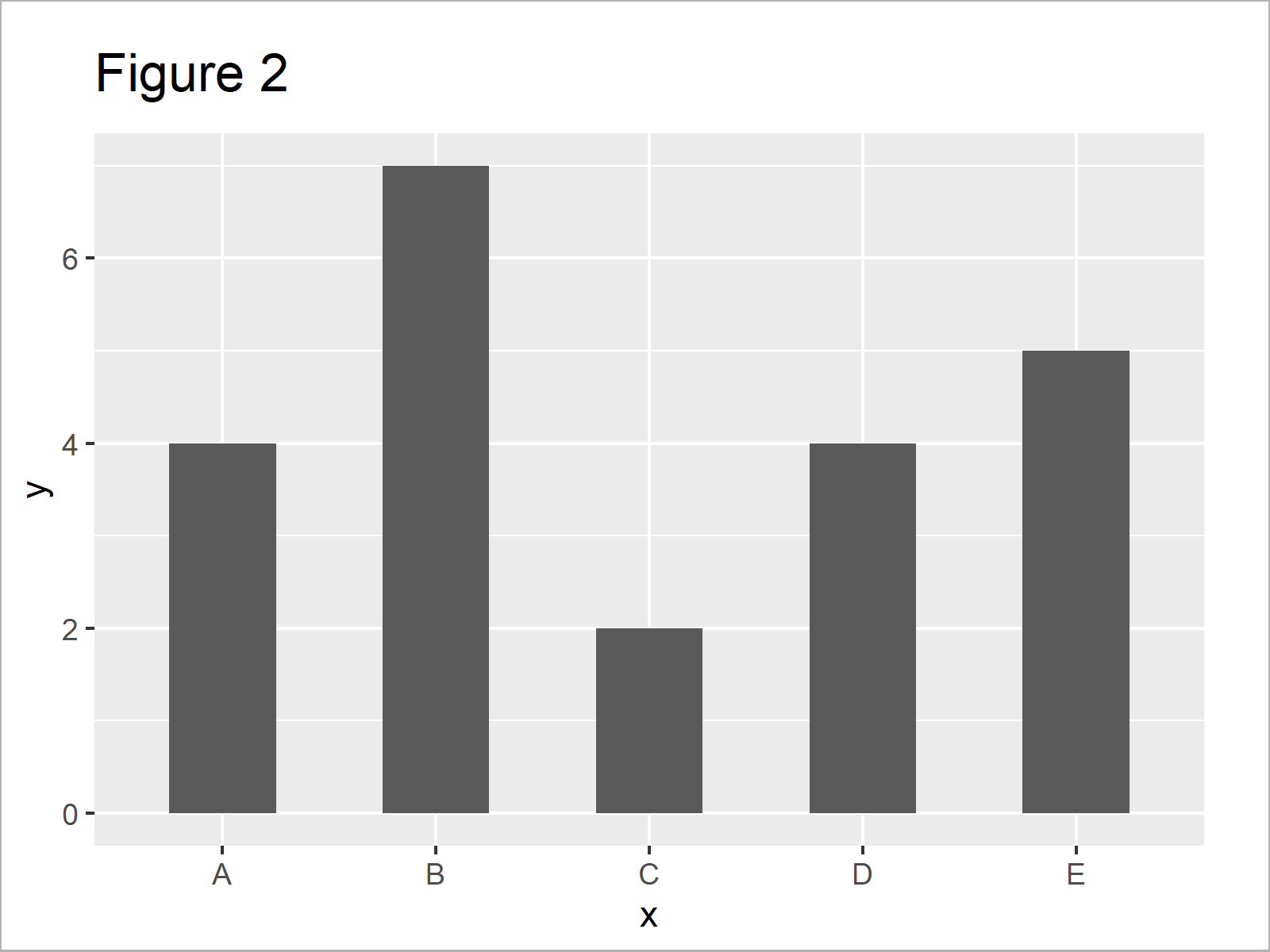# Change Space & Width of Bars in ggplot2 Barplot in R (2 Examples)

In this article, I’ll illustrate how to modify space and width of bars in a ggplot2 barchart in R programming.

Let’s do this!

## Example Data, Add-On Packages & Basic Graph

Have a look at the following example data:

```data <- data.frame(x = LETTERS[1:5],    # Create example data frame
y = c(4, 7, 2, 4, 5))
data                                    # Print example data frame```Have a look at the table that has been returned by the previous code. It shows that our example data consists of five rows and two variables.

The column x defines the groups (i.e. the bars) in our data. The column y specifies the height of each bar.

In this tutorial, we also need to install and load the ggplot2 package.

```install.packages("ggplot2")             # Install & load ggplot2
library("ggplot2")```

As next step, we can draw our data with default specifications of space and width using the following R code:

```ggplot(data, aes(x, y)) +               # Draw ggplot2 barchart with default width
geom_bar(stat = "identity")```Figure 1 shows the output of the previous R code: A ggplot2 bargraph with default specifications.

## Example 1: Decrease Width to Increase Space Between Bars in ggplot2 Barplot

Example 1 explains how to make the width of the bars in our ggplot2 barchart smaller to leave more space between the bars.

For this, we can use the width argument within the geom_bar function:

```ggplot(data, aes(x, y)) +               # Draw ggplot2 barchart with decreased width
geom_bar(stat = "identity",
width = 0.5)```In Figure 2 you can see that we have drawn a ggplot2 barplot with relatively thin bars and more empty space between the bars.

## Example 2: Increase Width to Decrease Space Between Bars in ggplot2 Barplot

In Example 2, I’ll show how to make the width of the bars larger to show only a very small area of space between the bars.

In contrast to Example 1, we have to increase the value assigned to the width argument within geom_bar function:

```ggplot(data, aes(x, y)) +               # Draw ggplot2 barchart with increased width
geom_bar(stat = "identity",
width = 0.99)```The output of the previous R programming code is shown in Figure 3: A ggplot2 barplot with less width between the bars.

Note that a width of 1 leads to bars that have no gap between each other anymore. Figure 3 basically shows the least possible space between bars of a barchart.

## Video, Further Resources & Summary

Do you need more explanations on the R syntax of this tutorial? Then I recommend watching the following video of my YouTube channel. I illustrate the R programming codes of this article in the video:

Please accept YouTube cookies to play this video. By accepting you will be accessing content from YouTube, a service provided by an external third party.If you accept this notice, your choice will be saved and the page will refresh.

Furthermore, you might read the related articles of my website.

In summary: In this tutorial you have learned how to increase and decrease the space between bars of a ggplot2 barplot in R. If you have further questions, please let me know in the comments. Furthermore, please subscribe to my email newsletter to get updates on the newest articles.

Subscribe to the Statistics Globe Newsletter